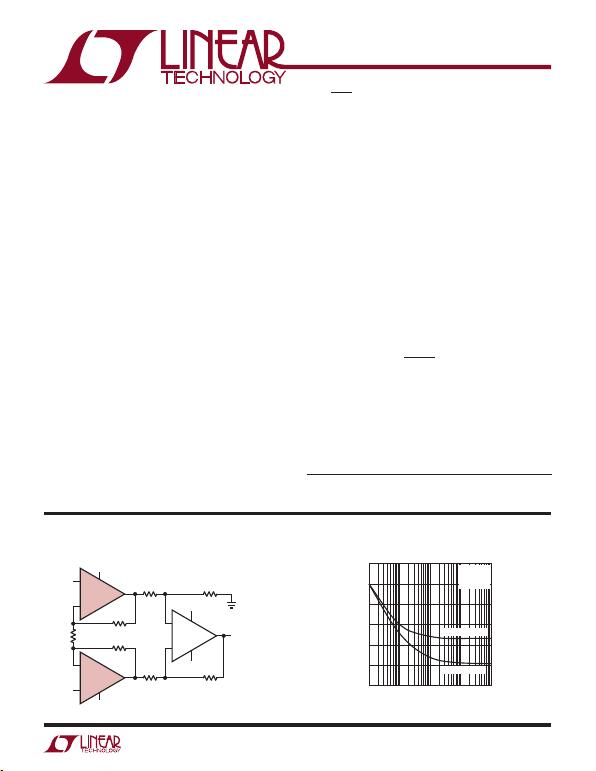1
623012fc
LT6230/LT6230-10
LT6231/LT6232
Typical applicaTion
FeaTures DescripTion
215MHz, Rail-to-Rail Output,
1.1nV/Hz, 3.5mA Op Amp Family
The LT
®
noise, rail-to-rail output unity-gain stable op amps that
feature 1.1nV/√Hz noise voltage and draw only 3.5mA of
supply current per ampliﬁer. These ampliﬁers combine
very low noise and supply current with a 215MHz gain-
bandwidth product, a 70V/µs slew rate and are optimized
for low supply voltage signal conditioning systems. The
LT6230-10 is a single ampliﬁer optimized for higher gain
applications resulting in higher gain bandwidth and slew
rate. The LT6230 and LT6230-10 include an enable pin
that can be used to reduce the supply current to less
than 10µA.
The ampliﬁer family has an output that swings within 50mV
of either supply rail to maximize the signal dynamic range
in low supply applications and is speciﬁed on 3.3V, 5V and
±5V supplies. The en ISUPPLY product of 1.9 per ampliﬁer
is among the most noise efﬁcient of any op amp.
The LT6230/LT6230-10 are available in the 6-lead SOT-23
package and the LT6231 dual is available in the 8-pin SO
package with standard pinouts. For compact layouts,
the dual is also available in a tiny dual ﬁne pitch leadless
package (DFN). The LT6232 is available in the 16-pin
SSOP package.
Low Noise Low Power Instrumentation Ampliﬁer
applicaTions
n Low Noise Voltage: 1.1nV/√Hz
n Low Supply Current: 3.5mA/Amp Max
n Low Offset Voltage: 350µV Max
n Gain Bandwidth Product:
LT6230: 215MHz; AV ≥ 1
LT6230-10: 1450MHz; AV ≥ 10
n Wide Supply Range: 3V to 12.6V
n Output Swings Rail-to-Rail
n Common Mode Rejection Ratio: 115dB Typ
n Output Current: 30mA
n Operating Temperature Range: –40°C to 85°C
n LT6230 Shutdown to 10µA Maximum
n LT6230/LT6230-10 in a Low Proﬁle (1mm)
ThinSOT™ Package
n Dual LT6231 in 8-Pin SO and Tiny DFN Packages
n LT6232 in a 16-Pin SSOP Package
n Ultrasound Ampliﬁers
n Low Noise, Low Power Signal Processing
n Active Filters
n Driving A/D Converters
n Rail-to-Rail Buffer Ampliﬁers
L, LT, LTC, LTM, Linear Technology and the Linear logo are registered trademarks of Linear
Technology Corporation. ThinSOT is a trademark of Linear Technology Corporation. All other
trademarks are the property of their respective owners.
+
R6
499Ω
LT6202
VS+
VOUT
IN+
IN+
1/2 LT6231
VS
VS+
+
1/2 LT6231
R7
499Ω
R4
499Ω
R2
196Ω
R1
10Ω
R3
196Ω R5
499Ω
623012 TA01a
AV = 40
BW = 5.1MHz
VS = ±1.5V to ±5V
IS = 10mA
EN = 5.8µVRMS INPUT REFERRED,
MEASUREMENT BW = 8MHz
VS
Noise Voltage and Unbalanced
Noise Current vs Frequency
FREQUENCY (Hz)
NOISE VOLTAGE (nV/√Hz)
6
5
4
3
2
1
010 1k 10k 100k
623012 TA01b
100
VS = ±2.5V
TA = 25°C
VCM = 0V
NOISE VOLTAGE
NOISE CURRENT
UNBALANCED NOISE CURRENT (pA/√Hz)
6
5
4
3
2
1
0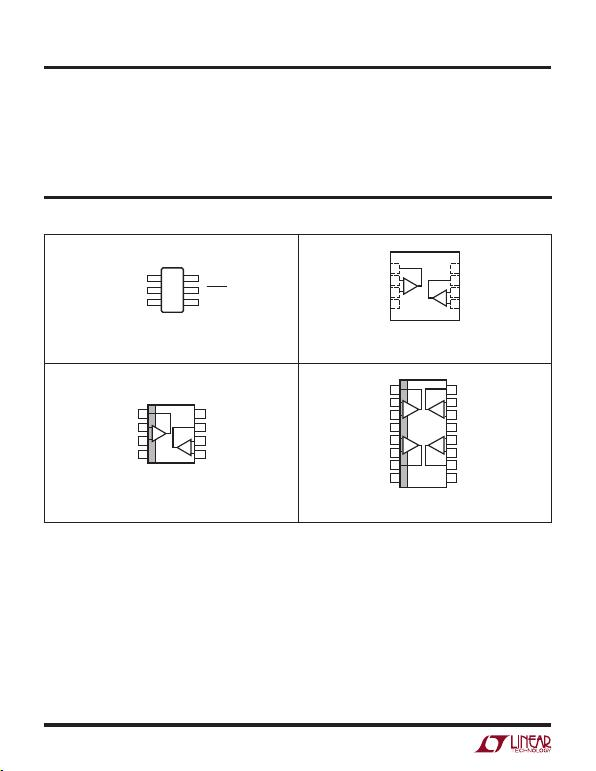LT6230/LT6230-10
LT6231/LT6232
2
623012fc
absoluTe MaxiMuM raTings
Total Supply Voltage (V+ to V) .............................. 12.6V
Input Current (Note 2) ......................................... ±40mA
Output Short-Circuit Duration (Note 3) ............ Indenite
Operating Temperature Range (Note 4)....40°C to 85°C
Speciﬁed Temperature Range (Note 5) ....40°C to 85°C
Junction Temperature ........................................... 150°C
(Note 1)
6 V+
5 ENABLE
4 –IN
OUT 1
TOP VIEW
S6 PACKAGE
V 2
+IN 3
TJMAX = 150°C, θJA = 250°C/W
TOP VIEW
DD PACKAGE
8-LEAD (3mm × 3mm) PLASTIC DFN
5
6
7
8
4
3
2
1OUT A
–IN A
+IN A
V
V+
OUT B
–IN B
+IN B
+
+
TJMAX = 125°C, θJA = 160°C/W
UNDERSIDE METAL CONNECTED TO V (PCB CONNECTION OPTIONAL)
TOP VIEW
V+
OUT B
–IN B
+IN B
OUT A
–IN A
+IN A
V
S8 PACKAGE
1
2
3
4
8
7
6
5
+
+
TJMAX = 150°C, θJA = 200°C/W
TOP VIEW
GN PACKAGE
1
2
3
4
5
6
7
8
16
15
14
13
12
11
10
9
OUT A
–IN A
+IN A
V+
+IN B
–IN B
OUT B
NC
OUT D
–IN D
+IN D
V
+IN C
–IN C
OUT C
NC
+
+
+
+
B C
TJMAX = 150°C, θJA = 135°C/W
pin conFiguraTion
Junction Temperature (DD Package) .................... 125°C
Storage Temperature Range .................. 65°C to 150°C
Storage Temperature Range
(DD Package) ........................................ 65°C to 125°C
Lead Temperature (Soldering, 10 sec) ...................300°C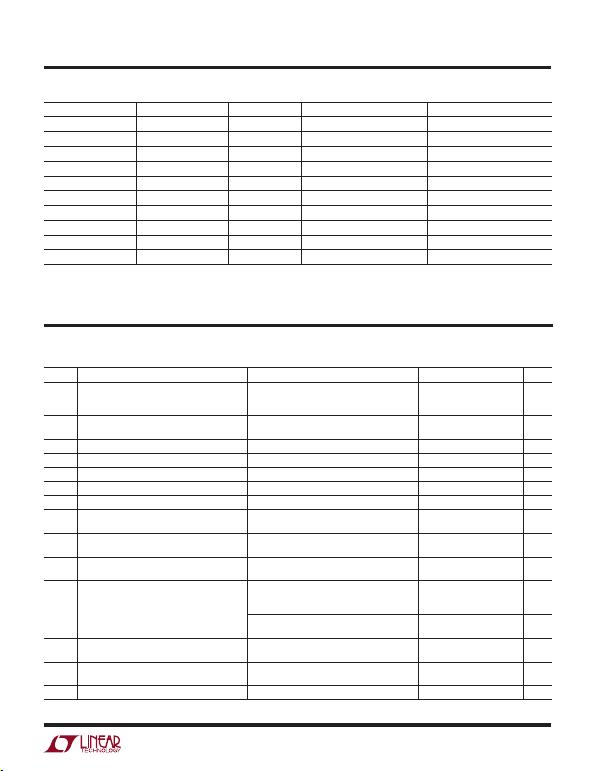3
623012fc
LT6230/LT6230-10
LT6231/LT6232
elecTrical characTerisTics
TA = 25°C, VS = 5V, 0V; VS = 3.3V, 0V; VCM = VOUT = half supply,
ENABLE = 0V, unless otherwise noted.
orDer inForMaTion
LEAD FREE FINISH TAPE AND REEL PART MARKING* PACKAGE DESCRIPTION SPECIFIED TEMPERATURE RANGE
LT6230CS6#PBF LT6230CS6#TRPBF LTAFJ 6-Lead Plastic TS0T-23 0°C to 70°C
LT6230IS6#PBF LT6230IS6#TRPBF LTAFJ 6-Lead Plastic TS0T-23 –40°C to 85°C
LT6230CS6-10#PBF LT6230CS6-10#TRPBF LTAFK 6-Lead Plastic TS0T-23 0°C to 70°C
LT6230IS6-10#PBF LT6230IS6-10#TRPBF LTAFK 6-Lead Plastic TS0T-23 –40°C to 85°C
LT6231CS8#PBF LT6230CS8#TRPBF 6231 8-Lead Plastic SO 0°C to 70°C
LT6231IS8#PBF LT6230IS8#TRPBF 6231I 8-Lead Plastic SO –40°C to 85°C
LT6231CDD#PBF LT6231CDD#TRPBF LAEU 8-Lead (3mm × 3mm) Plastic DFN 0°C to 70°C
LT6231IDD#PBF LT6231IDD#TRPBF LAEU 8-Lead (3mm × 3mm) Plastic DFN –40°C to 85°C
LT6232CGN#PBF LT6232CGN#TRPBF 6232 16-Lead Narrow Plastic SSOP 0°C to 70°C
LT6232IGN#PBF LT6232IGN#TRPBF 6232I 16-Lead Narrow Plastic SSOP –40°C to 85°C
Consult LTC Marketing for parts speciﬁed with wider operating temperature ranges. *The temperature grade is identiﬁed by a label on the shipping container.
Consult LTC Marketing for information on non-standard lead based ﬁnish parts.
SYMBOL PARAMETER CONDITIONS MIN TYP MAX UNITS
VOS Input Offset Voltage LT6230S6, LT6230S6-10
LT6231S8, LT6232GN
LT6231DD
100
50
75
500
350
450
µV
µV
µV
Input Offset Voltage Match
(Channel-to-Channel) (Note 6) 100 600 µV
IBInput Bias Current 5 10 µA
IB Match (Channel-to-Channel) (Note 6) 0.1 0.9 µA
IOS Input Offset Current 0.1 0.6 µA
Input Noise Voltage 0.1Hz to 10Hz 180 nVP-P
enInput Noise Voltage Density f = 10kHz, VS = 5V 1.1 1.7 nV/√Hz
in Input Noise Current Density, Balanced Source
Input Noise Current Density, Unbalanced Source f = 10kHz, VS = 5V, RS = 10k
f = 10kHz, VS = 5V, RS = 10k 1
2.4 pA/√Hz
pA/√Hz
Input Resistance Common Mode
Differential Mode 6.5
7.5
CIN Input Capacitance Common Mode
Differential Mode 2.9
7.7 pF
pF
AVOL Large-Signal Gain VS = 5V, VO = 0.5V to 4.5V, RL = 10k to VS/2
VS = 5V, VO = 0.5V to 4.5V, RL = 1k to VS/2
VS = 5V, VO = 1V to 4V, RL = 100Ω to VS/2
105
21
5.4
200
40
9
V/mV
V/mV
V/mV
VS = 3.3V, VO = 0.65V to 2.65V, RL = 10k to VS/2
VS = 3.3V, VO = 0.65V to 2.65V, RL = 1k to VS/2 90
16.5 175
32 V/mV
V/mV
VCM Input Voltage Range Guaranteed by CMRR, VS = 5V, 0V
Guaranteed by CMRR, VS = 3.3V, 0V 1.5
1.15 4
2.65 V
V
CMRR Common Mode Rejection Ratio VS = 5V, VCM = 1.5V to 4V
VS = 3.3V, VCM = 1.15V to 2.65V 90
90 115
115 dB
dB
CMRR Match (Channel-to-Channel) (Note 6) VS = 5V, VCM = 1.5V to 4V 84 120 dB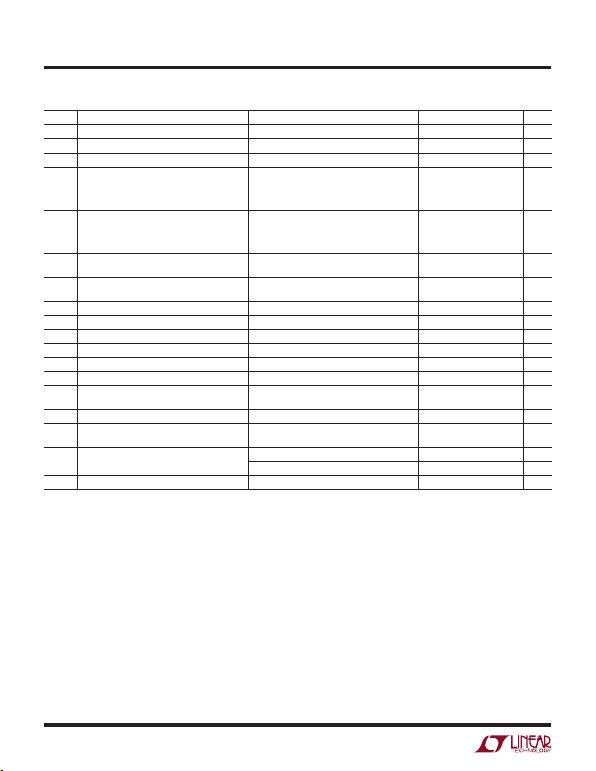LT6230/LT6230-10
LT6231/LT6232
4
623012fc
elecTrical characTerisTics
TA = 25°C, VS = 5V, 0V; VS = 3.3V, 0V; VCM = VOUT = half supply,
ENABLE = 0V, unless otherwise noted.
SYMBOL PARAMETER CONDITIONS MIN TYP MAX UNITS
PSRR Power Supply Rejection Ratio VS = 3V to 10V 90 115 dB
PSRR Match (Channel-to-Channel) (Note 6) VS = 3V to 10V 84 115 dB
Minimum Supply Voltage (Note 7) 3 V
VOL Output Voltage Swing Low (Note 8) No Load
ISINK = 5mA
VS = 5V, ISINK = 20mA
VS = 3.3V, ISINK = 15mA
4
85
240
185
40
190
460
350
mV
mV
mV
mV
VOH Output Voltage Swing High (Note 8) No Load
ISOURCE = 5mA
VS = 5V, ISOURCE = 20mA
VS = 3.3V, ISOURCE = 15mA
5
90
325
250
50
200
600
400
mV
mV
mV
mV
ISC Short-Circuit Current VS = 5V
VS = 3.3V ±30
±25 ±45
±40 mA
mA
ISSupply Current per Ampliﬁer
Disabled Supply Current per Ampliﬁer
ENABLE = V+ – 0.35V 3.15
0.2 3.5
10 mA
µA
IENABLE ENABLE Pin Current ENABLE = 0.3V –25 –75 µA
VLENABLE Pin Input Voltage Low 0.3 V
VHENABLE Pin Input Voltage High V+ – 0.35V V
Output Leakage Current ENABLE = V+ – 0.35V, VO = 1.5V to 3.5V 0.2 10 µA
tON Turn-On Time ENABLE = 5V to 0V, RL = 1k, VS = 5V 300 ns
tOFF Turn-Off Time ENABLE = 0V to 5V, RL = 1k, VS = 5V 41 µs
GBW Gain-Bandwidth Product Frequency = 1MHz, VS = 5V
LT6230-10 200
1300 MHz
MHz
SR Slew Rate VS = 5V, A
V = –1, RL = 1k, VO = 1.5V to 3.5V 42 60 V/µs
LT6230-10, VS = 5V, AV = –10, RL = 1k,
VO = 1.5V to 3.5V 250 V/µs
FPBW Full-Power Bandwidth VS = 5V, VOUT = 3VP-P (Note 9) 4.8 6.3 MHz
LT6230-10, HD2 = HD3 = ≤1% 11 MHz
tSSettling Time (LT6230, LT6231, LT6232) 0.1%, VS = 5V, VSTEP = 2V, AV = –1, RL = 1k 55 ns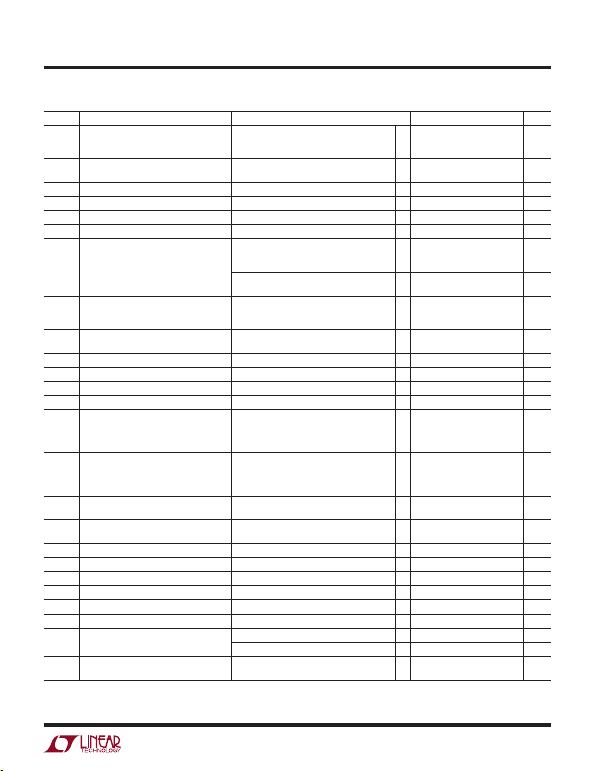5
623012fc
LT6230/LT6230-10
LT6231/LT6232
SYMBOL PARAMETER CONDITIONS MIN TYP MAX UNIT
VOS Input Offset Voltage LT6230CS6, LT6230CS6-10
LT6231CS8, LT6232CGN
LT6231CDD
l
l
l
600
450
550
µV
µV
µV
Input Offset Voltage Match
(Channel-to-Channel) (Note 6)
l800 µV
VOS TC Input Offset Voltage Drift (Note 10) VCM = Half Supply l0.5 3 µV/°C
IBInput Bias Current l11 µA
IB Match (Channel-to-Channel) (Note 6) l1 µA
IOS Input Offset Current l0.7 µA
AVOL Large-Signal Gain VS = 5V, VO = 0.5V to 4.5V, RL = 10k to VS/2
VS = 5V, VO = 0.5V to 4.5V, RL = 1k to VS/2
VS = 5V, VO = 1V to 4V, RL = 100Ω to VS/2
l
l
l
78
17
4.1
V/mV
V/mV
V/mV
VS = 3.3V, VO = 0.65V to 2.65V, RL = 10k to VS/2
VS = 3.3V, VO = 0.65V to 2.65V, RL = 1k to VS/2
l
l
66
13 V/mV
V/mV
VCM Input Voltage Range Guaranteed by CMRR
VS = 5V, 0V
Vs = 3.3V, 0V
l
l
1.5
1.15
4
2.65
V
V
CMRR Common Mode Rejection Ratio VS = 5V, VCM = 1.5V to 4V
VS = 3.3V, VCM = 1.15V to 2.65V
l
l
90
85 dB
dB
CMRR Match (Channel-to-Channel) (Note 6) VS = 5V, VCM = 1.5V to 4V l84 dB
PSRR Power Supply Rejection Ratio VS = 3V to 10V l85 dB
PSRR Match (Channel-to-Channel) (Note 6) VS = 3V to 10V l79 dB
Minimum Supply Voltage (Note 7) l3 V
VOL Output Voltage Swing Low (Note 8) No Load
ISINK = 5mA
VS = 5V, ISINK = 20mA
VS = 3.3V, ISINK = 15mA
l
l
l
l
50
200
500
380
mV
mV
mV
mV
VOH Output Voltage Swing High (Note 8) No Load
ISOURCE = 5mA
VS = 5V, ISOURCE = 20mA
VS = 3.3V, ISOURCE = 15mA
l
l
l
l
60
215
650
430
mV
mV
mV
mV
ISC Short-Circuit Current VS = 5V
VS = 3.3V
l
l
±25
±20 mA
mA
ISSupply Current per Ampliﬁer
Disabled Supply Current per Ampliﬁer
ENABLE = V+ – 0.25V
l
l
14.2 mA
µA
IENABLE ENABLE Pin Current ENABLE = 0.3V l–85 µA
VLENABLE Pin Input Voltage Low l0.3 V
VHENABLE Pin Input Voltage High lV+ – 0.25V V
Output Leakage Current ENABLE = V+ – 0.25V, VO = 1.5V to 3.5V l1 µA
tON Turn-On Time ENABLE = 5V to 0V, RL = 1k, VS = 5V l300 ns
tOFF Turn-Off Time ENABLE = 0V to 5V, RL = 1k, VS = 5V l65 µs
SR Slew Rate VS = 5V, AV = –1, RL = 1k, VO = 1.5V to 3.5V l35 V/µs
LT6230-10, AV = –10, RL = 1k, VO = 1.5V to 3.5V l225 V/µs
FPBW Full-Power Bandwidth (Note 9) VS = 5V, VOUT = 3VP-P; LT6230C, LT6231C,
LT6232C
l3.7 MHz
elecTrical characTerisTics
The l denotes the speciﬁcations which apply over the 0°C < TA < 70°C
temperature range. VS = 5V, 0V; VS = 3.3V, 0V; VCM = VOUT = half supply, ENABLE = 0V, unless otherwise noted.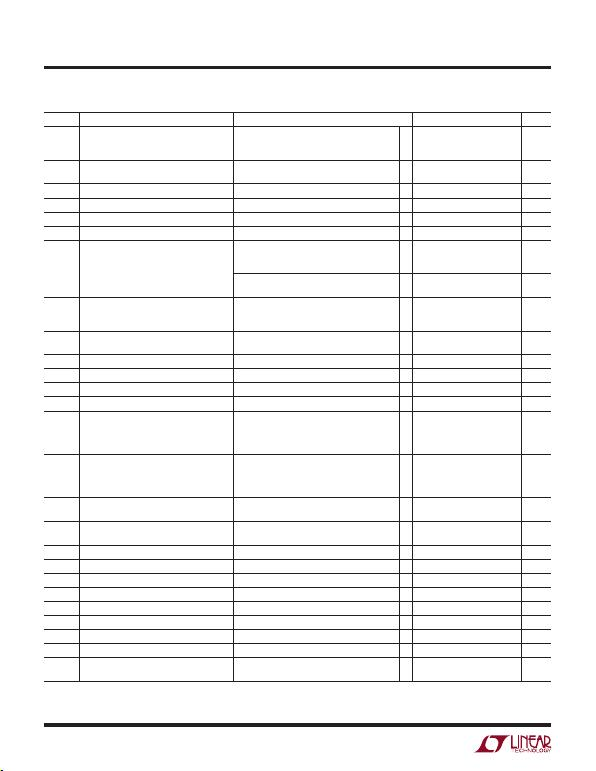LT6230/LT6230-10
LT6231/LT6232
6
623012fc
elecTrical characTerisTics
The l denotes the speciﬁcations which apply over the –40°C < TA < 85°C
temperature range. VS = 5V, 0V; VS = 3.3V, 0V; VCM = VOUT = half supply, ENABLE = 0V, unless otherwise noted. (Note 5)
SYMBOL PARAMETER CONDITIONS MIN TYP MAX UNITS
VOS Input Offset Voltage LT6230IS6, LT6230IS6-10
LT6231IS8, LT6232IGN
LT6231IDD
l
l
l
700
550
650
µV
µV
µV
Input Offset Voltage Match
(Channel-to-Channel) (Note 6)
l1000 µV
VOS TC Input Offset Voltage Drift (Note 10) VCM = Half Supply l0.5 3 µV/°C
IBInput Bias Current l12 µA
IB Match (Channel-to-Channel) (Note 6) l1.1 µA
IOS Input Offset Current l0.8 µA
AVOL Large-Signal Gain VS = 5V, VO = 0.5V to 4.5V, RL = 10k to VS/2
VS = 5V, VO = 0.5V to 4.5V, RL = 1k to VS/2
VS = 5V, VO = 1V to 4V, RL = 100Ω to VS/2
l
l
l
72
16
3.6
V/mV
V/mV
V/mV
VS = 3.3V, VO = 0.65V to 2.65V, RL = 10k to VS/2
VS = 3.3V, VO = 0.65V to 2.65V, RL = 1k to VS/2
l
l
60
12 V/mV
V/mV
VCM Input Voltage Range Guaranteed by CMRR
VS = 5V, 0V
VS = 3.3V, 0V
l
l
1.5
1.15
4
2.65
V
V
CMRR Common Mode Rejection Ratio VS = 5V, VCM = 1.5V to 4V
VS = 3.3V, VCM = 1.15V to 2.65V
l
l
90
85 dB
dB
CMRR Match (Channel-to-Channel) (Note 6) VS = 5V, VCM = 1.5V to 4V l84 dB
PSRR Power Supply Rejection Ratio VS = 3V to 10V l85 dB
PSRR Match (Channel-to-Channel) (Note 6) VS = 3V to 10V l79 dB
Minimum Supply Voltage (Note 7) l3 V
VOL Output Voltage Swing Low (Note 8) No Load
ISINK = 5mA
VS = 5V, ISINK = 15mA
VS = 3.3V, ISINK = 15mA
l
l
l
l
60
210
510
390
mV
mV
mV
mV
VOH Output Voltage Swing High (Note 6) No Load
ISOURCE = 5mA
VS = 5V, ISOURCE = 20mA
VS = 3.3V, ISOURCE = 15mA
l
l
l
l
70
220
675
440
mV
mV
mV
mV
ISC Short-Circuit Current VS = 5V
VS = 3.3V
l
l
±15
±15 mA
mA
ISSupply Current per Ampliﬁer
Disabled Supply Current per Ampliﬁer
ENABLE = V+ – 0.2V
l
l
14.4 mA
µA
IENABLE ENABLE Pin Current ENABLE = 0.3V l–100 µA
VLENABLE Pin Input Voltage Low l0.3 V
VHENABLE Pin Input Voltage High lV+ – 0.2V V
Output Leakage Current ENABLE = V+ – 0.2V, VO = 1.5V to 3.5V l1 µA
tON Turn-On Time ENABLE = 5V to 0V, RL = 1k, VS = 5V l300 ns
tOFF Turn-Off Time ENABLE = 0V to 5V, RL = 1k, VS = 5V l72 µs
SR Slew Rate VS = 5V, AV = –1, RL = 1k, VO = 1.5V to 3.5V l31 V/µs
LT6230-10, AV = –10, RL = 1k, VO = 1.5V to 3.5V l185 V/µs
FPBW Full-Power Bandwidth (Note 9) VS = 5V, VOUT = 3VP-P; LT6230I, LT6231I,
LT6232I
l3.3 MHz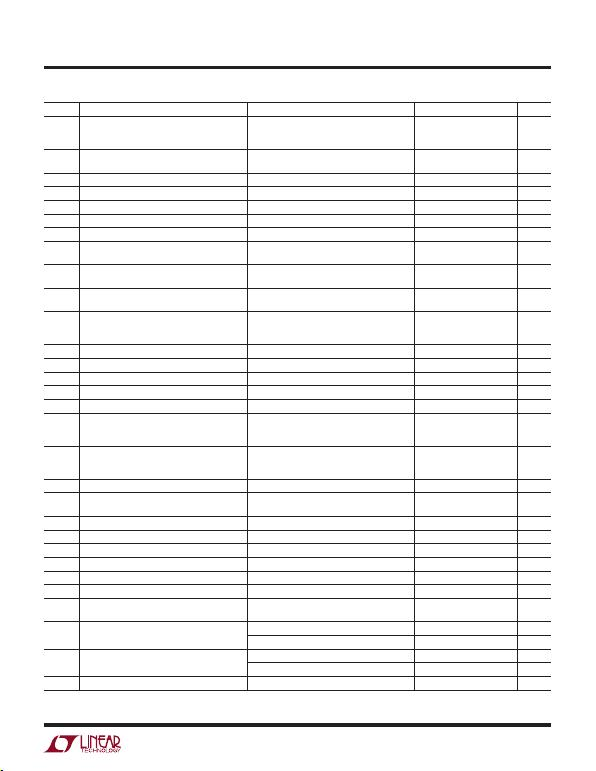7
623012fc
LT6230/LT6230-10
LT6231/LT6232
elecTrical characTerisTics
TA = 25°C, VS = ±5V, VCM = VOUT = 0V, ENABLE = 0V, unless otherwise noted.
SYMBOL PARAMETER CONDITIONS MIN TYP MAX UNITS
VOS Input Offset Voltage LT6230, LT6230-10
LT6231S8, LT6232GN
LT6231DD
100
50
75
500
350
450
µV
µV
µV
Input Offset Voltage Match
(Channel-to-Channel) (Note 6) 100 600 µV
IBInput Bias Current 5 10 µA
IB Match (Channel-to-Channel) (Note 6) 0.1 0.9 µA
IOS Input Offset Current 0.1 0.6 µA
Input Noise Voltage 0.1Hz to 10Hz 180 nVP-P
enInput Noise Voltage Density f = 10kHz 1.1 1.7 nV/√Hz
inInput Noise Current Density, Balanced Source
Input Noise Current Density, Unbalanced Source f = 10kHz, RS = 10k
f = 10kHz, RS = 10k 1
2.4 pA/√Hz
pA/√Hz
Input Resistance Common Mode
Differential Mode 6.5
7.5
CIN Input Capacitance Common Mode
Differential Mode 2.4
6.5 pF
pF
AVOL Large-Signal Gain VO = ±4.5V, RL = 10k
VO = ±4.5V, RL = 1k
VO = ±2V, RL = 100Ω
140
35
8.5
260
65
16
V/mV
V/mV
V/mV
VCM Input Voltage Range Guaranteed by CMRR –3 4 V
CMRR Common Mode Rejection Ratio VCM = –3V to 4V 95 120 dB
CMRR Match (Channel-to-Channel) (Note 6) VCM = –3V to 4V 89 125 dB
PSRR Power Supply Rejection Ratio VS = ±1.5V to ±5V 90 115 dB
PSRR Match (Channel-to-Channel) (Note 6) VS = ±1.5V to ±5V 84 115 dB
VOL Output Voltage Swing Low (Note 8) No Load
ISINK = 5mA
ISINK = 20mA
4
85
240
40
190
460
mV
mV
mV
VOH Output Voltage Swing High (Note 8) No Load
ISOURCE = 5mA
ISOURCE = 20mA
5
90
325
50
200
600
mV
mV
mV
ISC Short-Circuit Current ±30 mA
ISSupply Current per Ampliﬁer
Disabled Supply Current per Ampliﬁer
ENABLE = 4.65V 3.3
0.2 3.9 mA
µA
IENABLE ENABLE Pin Current ENABLE = 0.3V –35 –85 µA
VLENABLE Pin Input Voltage Low 0.3 V
VHENABLE Pin Input Voltage High 4.65 V
Output Leakage Current ENABLE = V+ – 4.65V, VO = ±1V 0.2 10 µA
tON Turn-On Time ENABLE = 5V to 0V, RL = 1k 300 ns
tOFF Turn-Off Time ENABLE = 0V to 5V, RL = 1k 62 µs
GBW Gain-Bandwidth Product Frequency = 1MHz
LT6230-10 150
1000 215
1450 MHz
MHz
SR Slew Rate AV = –1, RL = 1k, VO = –2V to 2V 50 70 V/µs
LT6230-10, AV = –10, RL = 1k, VO = –2V to 2V 320 V/µs
FPBW Full-Power Bandwidth VOUT = 3VP-P (Note 9) 5.3 7.4 MHz
LT6230-10, HD2 = HD3 ≤ 1% 11 MHz
tSSettling Time (LT6230, LT6231, LT6232) 0.1%, VSTEP = 2V, AV = –1, RL = 1k 50 ns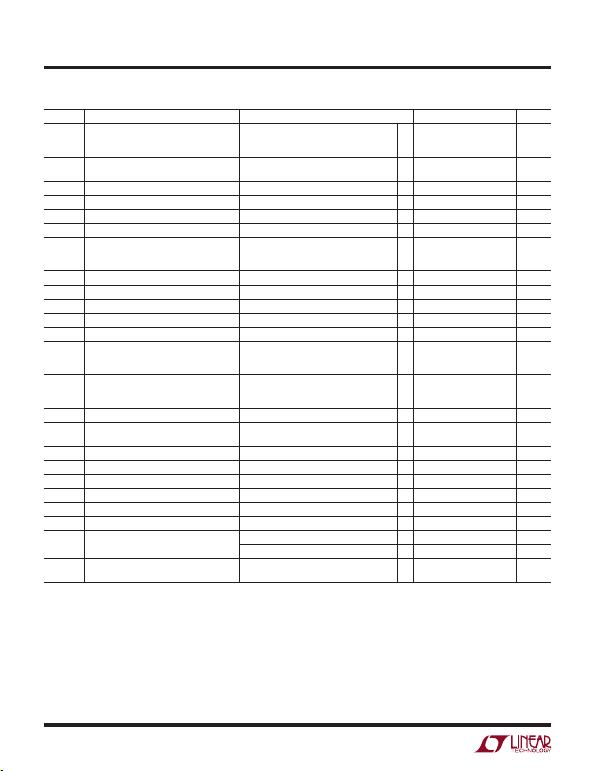LT6230/LT6230-10
LT6231/LT6232
8
623012fc
elecTrical characTerisTics
The l denotes the speciﬁcations which apply over the 0°C < TA < 70°C
temperature range. VS = ±5V, VCM = VOUT = 0V, ENABLE = 0V, unless otherwise noted.
SYMBOL PARAMETER CONDITIONS MIN TYP MAX UNITS
VOS Input Offset Voltage LT6230CS6, LT6230CS6-10
LT6231CS8, LT6232CGN
LT6231CDD
l
l
l
600
450
550
µV
µV
µV
Input Offset Voltage Match
(Channel-to-Channel) (Note 6)
l800 µV
VOS TC Input Offset Voltage Drift (Note 10) l0.5 3 µV/°C
IBInput Bias Current l11 µA
IB Match (Channel-to-Channel) (Note 6) l1 µA
IOS Input Offset Current l0.7 µA
AVOL Large-Signal Gain VO = ±4.5V, RL = 10k
VO = ±4.5V, RL = 1k
VO = ±2V, RL = 100Ω
l
l
l
100
27
6
V/mV
V/mV
V/mV
VCM Input Voltage Range Guaranteed by CMRR l–3 4 V
CMRR Common Mode Rejection Ratio VCM = –3V to 4V l95 dB
CMRR Match (Channel-to-Channel) (Note 6) VCM = –3V to 4V l89 dB
PSRR Power Supply Rejection Ratio VS = ±1.5V to ±5V l85 dB
PSRR Match (Channel-to-Channel) (Note 6) VS = ±1.5V to ±5V l79 dB
VOL Output Voltage Swing Low (Note 8) No Load
ISINK = 5mA
ISINK = 20mA
l
l
l
50
200
500
mV
mV
mV
VOH Output Voltage Swing High (Note 8) No Load
ISOURCE = 5mA
ISOURCE = 20mA
l
l
l
60
215
650
mV
mV
mV
ISC Short-Circuit Current l±25 mA
ISSupply Current per Ampliﬁer
Disabled Supply Current per Ampliﬁer
ENABLE = 4.75V
l
l
14.6 mA
µA
IENABLE ENABLE Pin Current ENABLE = 0.3V l–95 µA
VLENABLE Pin Input Voltage Low l0.3 V
VHENABLE Pin Input Voltage High l4.75 V
Output Leakage Current ENABLE = 4.75V, VO = ±1V l1 µA
tON Turn-On Time ENABLE = 5V to 0V, RL = 1k l300 ns
tOFF Turn-Off Time ENABLE = 0V to 5V, RL = 1k l85 µs
SR Slew Rate AV = –1, RL = 1k, VO = –2V to 2V l44 V/µs
LT6230-10, AV = –10, RL = 1k, VO = –2V to 2V l315 V/µs
FPBW Full-Power Bandwidth VOUT = 3VP-P (Note 9) LT6230C, LT6231C,
LT6232C
l4.66 MHz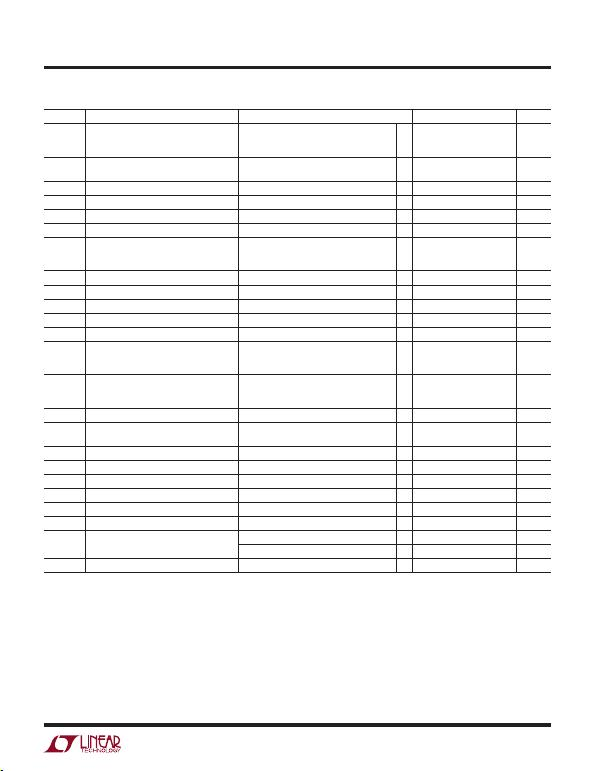9
623012fc
LT6230/LT6230-10
LT6231/LT6232
elecTrical characTerisTics
The l denotes the speciﬁcations which apply over the –40°C < TA < 85°C
temperature range. VS = ±5V, VCM = VOUT = 0V, ENABLE = 0V, unless otherwise noted. (Note 5)
SYMBOL PARAMETER CONDITIONS MIN TYP MAX UNITS
VOS Input Offset Voltage LT6230I, LT6230I-10
LT6231IS8, LT6232IGN
LT6231IDD
l
l
l
700
550
650
µV
µV
µV
Input Offset Voltage Match
(Channel-to-Channel) (Note 6)
l1000 µV
VOS TC Input Offset Voltage Drift (Note 10) l0.5 3 µV/°C
IBInput Bias Current l12 µA
IB Match (Channel-to-Channel) (Note 6) l1.1 µA
IOS Input Offset Current l0.8 µA
AVOL Large-Signal Gain VO = ±4.5V, RL = 10k
VO = ±4.5V, RL = 1k
VO = ±1.5V, RL = 100Ω
l
l
l
93
25
4.8
V/mV
V/mV
V/mV
VCM Input Voltage Range Guaranteed by CMRR l–3 4 V
CMRR Common Mode Rejection Ratio VCM = –3V to 4V l95 dB
CMRR Match (Channel-to-Channel) (Note 6) VCM = –3V to 4V l89 dB
PSRR Power Supply Rejection Ratio VS = ±1.5V to ±5V l85 dB
PSRR Match (Channel-to-Channel) (Note 6) VS = ±1.5V to ±5V l79 dB
VOL Output Voltage Swing Low (Note 8) No Load
ISINK = 5mA
ISINK = 15mA
l
l
l
60
210
510
mV
mV
mV
VOH Output Voltage Swing High (Note 8) No Load
ISOURCE = 5mA
ISOURCE = 20mA
l
l
l
70
220
675
mV
mV
mV
ISC Short-Circuit Current l±15 mA
ISSupply Current per Ampliﬁer
Disabled Supply Current per Ampliﬁer
ENABLE = 4.8V
l
l
14.85 mA
µA
IENABLE ENABLE Pin Current ENABLE = 0.3V l–110 µA
VLENABLE Pin Input Voltage Low l0.3 V
VHENABLE Pin Input Voltage High l4.8 V
Output Leakage Current ENABLE = 4.8V, VO = ±1V l1 µA
tON Turn-On Time ENABLE = 5V to 0V, RL = 1k l300 ns
tOFF Turn-Off Time ENABLE = 0V to 5V, RL = 1k l72 µs
SR Slew Rate AV = –1, RL = 1k, VO = –2V to 2V l37 V/µs
LT6230-10, AV = –10, RL = 1k, VO = –2V to 2V l260 V/µs
FPBW Full-Power Bandwidth (Note 9) VOUT = 3VP-P; LT6230I, LT6231I, LT6232I l3.9 MHz
Note 1: Stresses beyond those listed under Absolute Maximum Ratings
may cause permanent damage to the device. Exposure to any Absolute
Maximum Rating condition for extended periods may affect device
Note 2: Inputs are protected by back-to-back diodes. If the differential
input voltage exceeds 0.7V, the input current must be limited to less than
40mA.
Note 3: A heat sink may be required to keep the junction temperature
below the absolute maximum rating when the output is shorted
indeﬁnitely.
Note 4: The LT6230C/LT6230I the LT6231C/LT6231I, and LT6232C/LT6232I
are guaranteed functional over the temperature range of –40°C and 85°C.
Note 5: The LT6230C/LT6231C/LT6232C are guaranteed to meet speciﬁed
performance from 0°C to 70°C. The LT6230C/LT6231C/LT6232C are
designed, characterized and expected to meet speciﬁed performance from
–40°C to 85°C, but are not tested or QA sampled at these temperatures.
The LT6230I/LT6231I/LT6232I are guaranteed to meet specied
performance from –40°C to 85°C.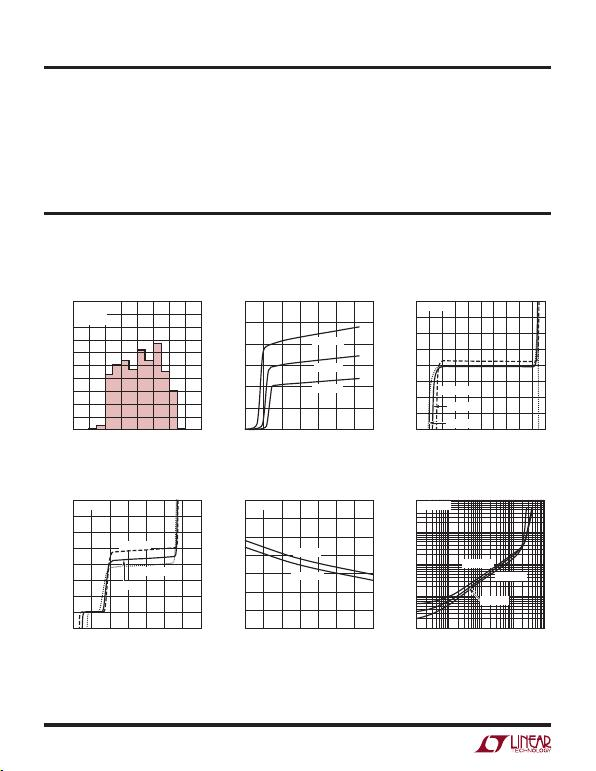LT6230/LT6230-10
LT6231/LT6232
10
623012fc
Note 6: Matching parameters are the difference between the two ampliﬁers
A and D and between B and C of the LT6232; between the two ampliﬁers
of the LT6231. CMRR and PSRR match are deﬁned as follows: CMRR and
PSRR are measured in µV/V on the matched ampliﬁers. The difference is
calculated between the matching sides in µV/V. The result is converted to
dB.
Note 7: Minimum supply voltage is guaranteed by power supply rejection
ratio test.
elecTrical characTerisTics
Note 8: Output voltage swings are measured between the output and
power supply rails.
Note 9: Full-power bandwidth is calculated from the slew rate:
FPBW = SR/2πVP
Note 10: This parameter is not 100% tested.
Typical perForMance characTerisTics
Input Bias Current
vs Common Mode Voltage Input Bias Current vs Temperature
Output Saturation Voltage
VOS Distribution
Supply Current vs Supply Voltage
(Per Ampliﬁer)
Offset Voltage vs Input Common
Mode Voltage
(LT6230/LT6231/LT6232)
INPUT OFFSET VOLTAGE (µV)
–200
0
NUMBER OF UNITS
10
20
30
40
–100 0 100 200
623012 GO1
50
100
90
80
70
60
–150 –50 50 150
VS = 5V, 0V
VCM = V+/2
S8
TOTAL SUPPLY VOLTAGE (V)
0
SUPPLY CURRENT (mA)
6
623012 GO2
2 4 8
6
5
4
3
2
1
010 12 14
TA = 125°C
TA = 25°C
TA = –55°C
INPUT COMMON MODE VOLTAGE (V)
0
OFFSET VOLTAGE (mV)
1.5
623012 GO3
0.5 1 2
2.0
1.5
1.0
0.5
0
–0.5
–1.0
–1.5
–2.0 3 4 52.5 3.5 4.5
TA = –55°C
VS = 5V, 0V
TA = 25°C
TA = 125°C
COMMON MODE VOLTAGE (V)
–1
INPUT BIAS CURRENT (µA)
2
623012 GO4
0 1 3
14
12
10
8
6
4
2
–2
0
456
TA = 125°C
TA = –55°C
TA = 25°C
VS = 5V, 0V
TEMPERATURE (°C)
–50
INPUT BIAS CURRENT (µA)
25
623012 GO5
–25 0 50
10
9
8
7
6
5
4
375 100 125
VCM = 4V
VCM = 1.5V
VS = 5V, 0V
0.01 0.1
0.001
OUTPUT SATURATION VOLTAGE (V)
0.01
10
1 10010
623012 GO6
0.1
1
VS = 5V, 0V
TA = –55°C
TA = 125°C
TA = 25°C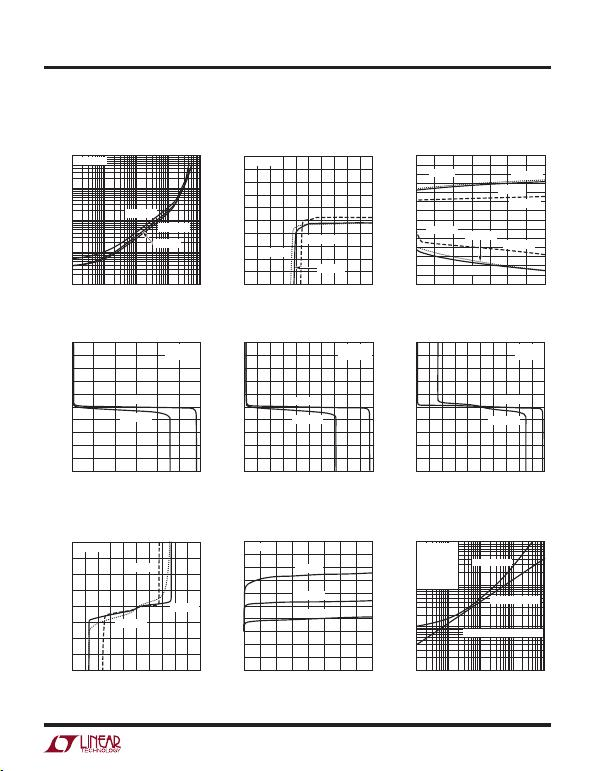11
623012fc
LT6230/LT6230-10
LT6231/LT6232
Typical perForMance characTerisTics
Open-Loop Gain Open-Loop Gain Open-Loop Gain
Offset Voltage vs Output Current Warm-Up Drift vs Time
Total Noise vs Total Source
Resistance
Output Saturation Voltage
vs Load Current (Output High) Minimum Supply Voltage
Output Short-Circuit Current
vs Power Supply Voltage
(LT6230/LT6231/LT6232)
OUTPUT SATURATION VOLTAGE (V)
623012 G07
0.01 0.1
0.01
10
1 10010
0.001
0.1
1
VS = 5V, 0V
TA = –55°C
TA = 125°C
TA = 25°C
TOTAL SUPPLY VOLTAGE (V)
0
OFFSET VOLTAGE (mV)
1.5
623012 G08
0.5 1 2
1.0
0.8
0.6
0.4
0.2
0
–0.2
–0.4
–0.6
–0.8
–1.0 3 4 52.5 3.5 4.5
TA = –55°C
TA = 125°C
TA = 25°C
VCM = VS/2
POWER SUPPLY VOLTAGE (±V)
1.5
OUTPUT SHORT-CIRCUIT CURRENT (mA)
3
623012 GO9
2 2.5 3.5
70
60
40
20
50
30
10
0
–20
–40
–70
–60
–10
–30
–50
4 4.5 5
TA = 125°C
TA = –55°C
TA = –55°C
TA = 25°C
SINKING
SOURCING
TA = 25°C
TA = 125°C
OUTPUT VOLTAGE (V)
0
INPUT VOLTAGE (mV)
1.5
623012 G10
0.5 1 2
2.5
2.0
1.5
1.0
0.5
0
–0.5
–1.0
–1.5
–2.0
–2.5 32.5
RL = 100Ω
RL = 1k
VS = 3V, 0V
TA = 25°C
OUTPUT VOLTAGE (V)
0
INPUT VOLTAGE (mV)
1.5
623012 G11
0.5 1 2
0
3 4 52.5 3.5 4.5
RL = 100Ω
RL = 1k
VS = 5V, 0V
TA = 25°C
2.5
2.0
1.5
1.0
0.5
–0.5
–1.0
–1.5
–2.0
–2.5
OUTPUT VOLTAGE (V)
–5
INPUT VOLTAGE (mV)
–2
623012 G12
–4 –3 –1
0
135024
RL = 100Ω
RL = 1k
VS = ±5V
TA = 25°C
2.5
2.0
1.5
1.0
0.5
–0.5
–1.0
–1.5
–2.0
–2.5
OUTPUT CURRENT (mA)
–75
OFFSET VOLTAGE (mV)
623012 G13
–45 –15
2.0
1.5
1.0
0.5
0
–0.5
–1.0
–1.5
–2.0 0 30 7560–60 –30 15 45
TA = –55°C
TA = 125°C
VS = ±5V
TA = 25°C
TIME AFTER POWER-UP (s)
0
CHANGE IN OFFSET VOLTAGE (µV)
60
623012 G14
20 100
30
28
24
20
16
26
22
18
14
12
10 140
40 80 120 160
TA = 25°C
VS = ±5V
VS = ±2.5V
VS = ±1.5V
SOURCE RESISTANCE (Ω)
1
TOTAL NOISE (nV/√Hz)
10
10 1k 10k 100k
623012 G15
0.1 100
100 VS = ±2.5V
VCM = 0V
f = 100kHz
UNBALANCED
SOURCE
RESISTORS
TOTAL NOISE
RESISTOR NOISE
AMPLIFIER NOISE VOLTAGE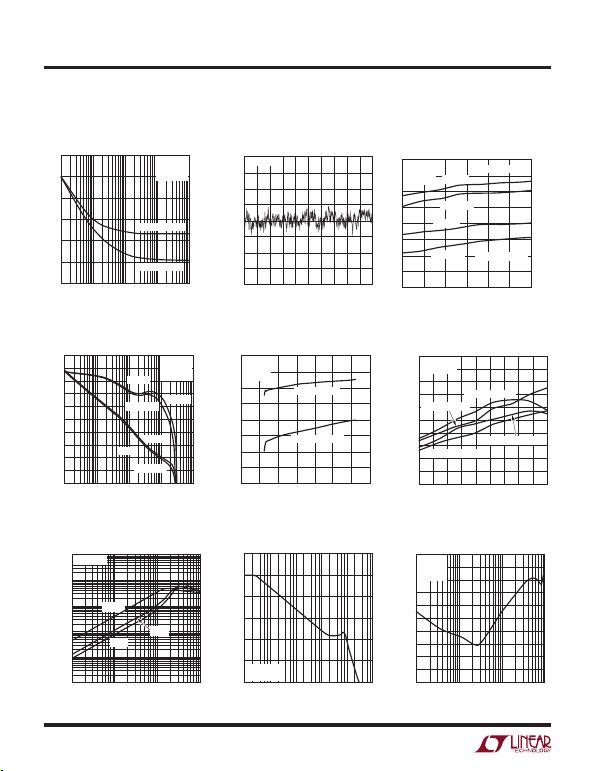LT6230/LT6230-10
LT6231/LT6232
12
623012fc
Typical perForMance characTerisTics
Open-Loop Gain vs Frequency
Gain Bandwidth and Phase
Margin vs Supply Voltage Slew Rate vs Temperature
Output Impedance vs Frequency
Common Mode Rejection Ratio
vs Frequency Channel Separation vs Frequency
Noise Voltage and Unbalanced
Noise Current vs Frequency
0.1Hz to 10Hz Output Voltage
Noise
Gain Bandwidth and Phase
Margin vs Temperature
(LT6230/LT6231/LT6232)
FREQUENCY (Hz)
NOISE VOLTAGE (nV/√Hz)
6
5
4
3
2
1
010 1k 10k 100k
623012 G16
100
VS = ±2.5V
TA = 25°C
VCM = 0V
NOISE VOLTAGE
NOISE CURRENT
UNBALANCED NOISE CURRENT (pA/√Hz)
6
5
4
3
2
1
0
5s/DIV
623012 G17
100nV
100nV/DIV
–100nV
VS = ±2.5V
TEMPERATURE (°C)
–55
GAIN BANDWIDTH (MHz)
5
623012 G18
–25 35
240
220
200
180
140
160
PHASE MARGIN (DEG)
70
60
50
40
65 95 125
VS = ±5V
VS = 3V, 0V
VS = ±5V
VS = 3V, 0V
PHASE MARGIN
GAIN BANDWIDTH
CL = 5pF
RL = 1k
VCM = VS/2
FREQUENCY (Hz)
GAIN (dB)
80
70
50
30
0
–10
60
40
10
20
–20
PHASE (dB)
120
100
60
20
–60
80
40
–20
–40
0
–80
100k 10M 100M 1G
623012 G19
1M
CL = 5pF
RL = 1k
VCM = VS/2
PHASE
GAIN
VS = ±5V
VS = 3V, 0V
VS = ±5V
VS = 3V, 0V
TOTAL SUPPLY VOLTAGE (V)
0
GAIN BANDWIDTH (MHz)
6
623012 G20
2 4 8
220
240
200
180
140
160
PHASE MARGIN (DEG)
70
60
50
40
10 12 14
PHASE MARGIN
GAIN BANDWIDTH
TA = 25°C
CL = 5pF
RL = 1k
TEMPERATURE (°C)
–55
SLEW RATE (V/µs)
5
623012 G21
–35 –15 45
90
100
110
120
80
70
50
20
30
60
40
8525 65 105 125
VS = ±5V FALLING
VS = ±2.5V RISING
AV = –1
RF = RG = 1k
VS = ±5V RISING
VS = ±2.5V FALLING
FREQUENCY (Hz)
1
OUTPUT IMPEDANCE (Ω)
10
100k 10M 100M
623012 G22
0.01
0.1
1M
1k
100
VS = 5V, 0V
AV = 10
AV = 1
AV = 2
FREQUENCY (Hz)
20
COMMON MODE REJECTION RATIO (dB)
40
60
80
120
100
10k 100M100k 1G10M
623012 G23
01M
VS = 5V, 0V
VCM = VS/2
FREQUENCY (Hz)
100k
CHANNEL SEPARATION (dB)
–40
–50
–60
–70
–80
–90
–100
–110
–120
–130
–140
1M 10M 100M
623012 G24
AV = 1
TA = 25°C
VS = ±5V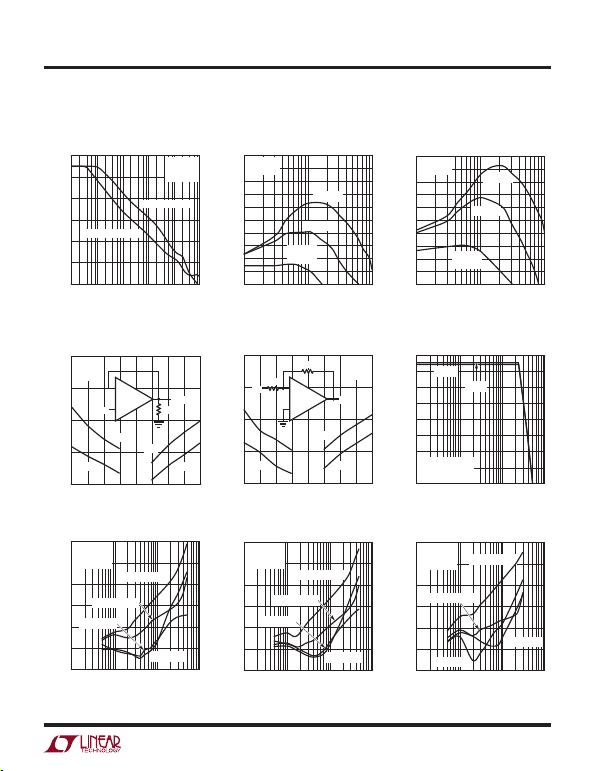13
623012fc
LT6230/LT6230-10
LT6231/LT6232
Typical perForMance characTerisTics
Settling Time vs Output Step
(Noninverting)
Settling Time vs Output Step
(Inverting)
Maximum Undistorted Output
Signal vs Frequency
Distortion vs Frequency Distortion vs Frequency Distortion vs Frequency
Power Supply Rejection Ratio
vs Frequency
Series Output Resistance and
Series Output Resistance and
(LT6230/LT6231/LT6232)
FREQUENCY (Hz)
20
POWER SUPPLY REJECTION RATIO (dB)
40
60
80
120
100
1k 10k 100M100k 10M
623012 G25
01M
VS = 5V, 0V
TA = 25°C
VCM = VS/2
NEGATIVE SUPPLY
POSITIVE SUPPLY
10
OVERSHOOT (%)
50
45
40
35
30
25
20
15
10
5
0100 1000
623012 G26
VS = 5V, 0V
AV = 1
RS = 10Ω
RS = 20Ω
RS = 50Ω
RL = 50Ω
10
OVERSHOOT (%)
50
45
40
35
30
25
20
15
10
5
0100 1000
623012 G27
VS = 5V, 0V
AV = 2
RS = 10Ω
RS = 20Ω
RS = 50Ω
RL = 50Ω
OUTPUT STEP (V)
–4
SETTLING TIME (ns)
0
623012 G28
–3 –2 –1 1
100
200
150
50
0234
1mV
10mV
1mV
10mV
VS = ±5V
TA = 25°C
AV = 1
+
500Ω
VOUT
VIN
OUTPUT STEP (V)
–4
SETTLING TIME (ns)
0
623012 G29
–3 –2 –1 1
200
150
0
50
100
234
1mV
10mV
1mV
10mV
VS = ±5V
TA = 25°C
AV = –1
+
500Ω
500Ω
VOUT
VIN
FREQUENCY (Hz)
10k
OUTPUT VOLTAGE SWING (VP-P)
10
9
8
7
6
5
4
3
2
100k 1M 10M
623012 G30
VS = ±5V
TA = 25°C
HD2, HD3 < –40dBc
AV = –1
AV = 2
FREQUENCY (Hz)
10k
DISTORTION (dBc)
–40
–50
–60
–70
–80
–90
–100
100k 1M 10M
623012 G31
VS = ±2.5V
AV = 1
VOUT = 2VP-P
RL = 100Ω, 3RD
RL = 1k, 3RD
RL = 100Ω, 2ND
RL = 1k, 2ND
FREQUENCY (Hz)
10k
DISTORTION (dBc)
–40
–50
–60
–70
–80
–90
–100
100k 1M 10M
623012 G32
VS = ±5V
AV = 1
VOUT = 2VP-P
RL = 100Ω, 3RD
RL = 1k, 3RD
RL = 1k, 2ND
RL = 100Ω, 2ND
FREQUENCY (Hz)
10k
DISTORTION (dBc)
–40
–50
–60
–70
–80
–90
–100
100k 1M 10M
623012 G33
VS = ±2.5V
AV = 2
VOUT = 2VP-P RL = 100Ω, 3RD
RL = 1k, 3RD
RL = 1k, 2ND
RL = 100Ω, 2ND